Frictional Properties | Textile Study Center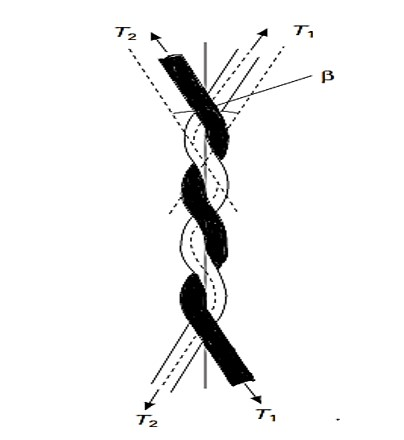# Frictional Properties

2.14K
0#### Md Sohanur Rahman Sobuj

Co-founder , Admin & Author at Textile Study Center
Student of Bangladesh University of Textiles (BUTEX) Department: Apparel Engineering## Frictional Properties

Friction is a vital important factor in the application of fibrous materials to engineering. Frictional property is the surface property of the fibers when two solid surfaces slide against each other. Due to fiber friction, static charge and heat energy is produced.

Friction:

Friction is the force of two surfaces in contact, or the force of a medium acting on a moving object.

❶  Fiber friction that is in between two same or different fibers.

❷  Fiber Solid friction that is present in between fiber and solid particle or surface.

Importance of fiber friction:

Friction of fabrics is important from different viewpoints:

⦿ Friction of fabrics against skin is an important aspect of comfort and

⦿ Fabric friction is important in appearance control of the fabric

⦿ Frictional force holds the fibers in a sliver and the interlacing threads in a fabric.

⦿ If the friction is too low, the yarn strength will be reduced.

⦿ If the friction is too low, the dimensional stability of cloth will be reduced.

Problem of friction in textile:

⦿ Friction causes nap formation.

⦿ Friction increase yarn hairiness.

⦿ Friction worn out parts of the machine.

⦿ Fiber friction can lead to damage of fiber surface.

⦿ High friction causes low handle properties of fabric.

⦿ Friction at guides and pulleys can lead to serious problems during yarn passing.

⦿ In high speed machine-sewing frictional heating can result in damage, both to the needle and to the fabric.

Types of friction:

There are two types of frictions

Static Friction: Static friction is the force that must be overcome to begin sliding of two objects or fibers in contact. It is independent of the area of contact. Static frictional force is equal and opposite to the applied loading force up to a maximum force and no acceleration or movement occurs below the maximum force. According to the Amonton’s law, static frictional force is proportional to the normal force pushing two surfaces together: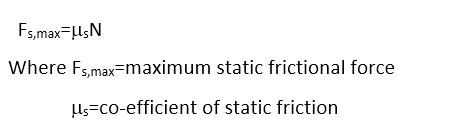Kinetic or Dynamic friction: Kinetic friction is the force that must be overcome to continue sliding. For most materials, the kinetic friction co-efficient is independent of the sliding speed and is smaller than the static friction co-efficient. Kinetic frictional force is opposite to the direction of motion and is also proportional to the normal force: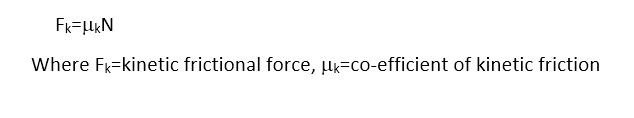Amonton’s law of friction

Leonardo da Vinci (1452-1519) proposed two basic laws of sliding friction of solids:

❶  Friction is independent of the area of the solids.

❷  Friction is directly proportional to the load that is normal to the sliding direction.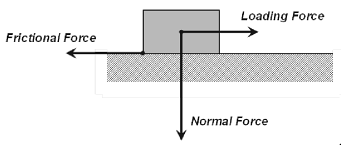These two laws were later rediscovered by Amonton’s in 1699 and are expressed mathematically. So, Amonton’s law: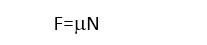Where, F=frictional force, N=normal force (load), m=coefficient of friction

The Amonton’s law for a yarn passing round a guide can be expressed in the form: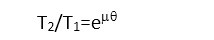WhereT1=incoming tension, T2=leaving tension,q=angle of contact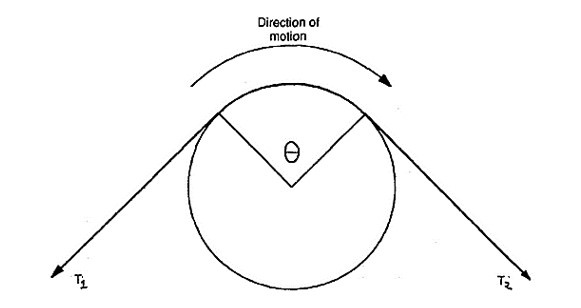Co-efficient of friction:

The ratio between frictional force and normal force is constant and this value is called the co-efficient of friction. Co-efficient of friction is independent of sliding speed.Method for measuring co-efficient of friction:

1. Capstan method
2. Buckle & Pollitt’s method
3. Abboh & Grasberg method
4. Gutheric & Oliver’s method

Capstan method: It is the most commonly used to measure the co-efficient of friction. Capstan method are two types:

❶  Static capstan method

❷  Dynamic capstan method

Static capstan method:

A static form of the method is illustrated in Fig. (a). In this method, a loop of yarn is placed over the guide and a small load placed on one side. The load on the other side is then decreased until slippage commences. If the yarn incoming tension (T1), leaving tension (T2), angle(q) of those tension(T2>T1) are measured and the coefficient of friction is calculated from the Amonton’s law, according to the following equation: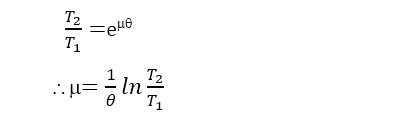Dynamic capstan method:

A dynamic form of the method is illustrated in Fig. (b). In this method, a yarn is running continuously over the guide. If the yarn incoming tension (T1), leaving tension (T2), angle (q) of those tension (T2>T1) are measured and the coefficient of friction is calculated the from Amonton’s law, according to the following equation: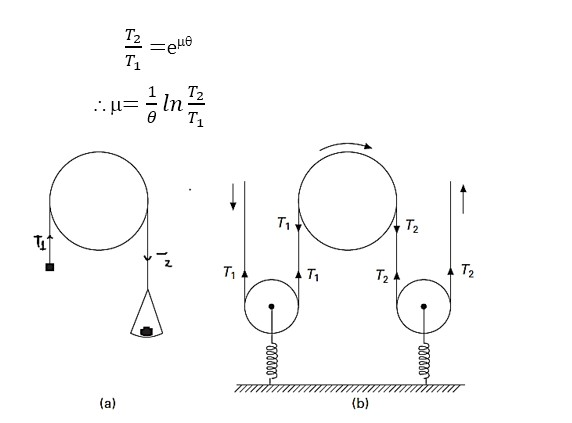Measurement of inter-fiber friction:

For the measurement of inter-fiber friction, Lindberg and Gralén introduced a method in which the two fibers are twisted together as shown in Fig. If the difference between the tensions applied to the opposite ends of each fiber is increased, the fibers will eventually slip over one another. The coefficient of fiber-to-fiber friction is determined using the Amonton’s law. It is shown that: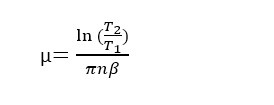Where T2 and T1 are the tensions in the fibers, n is the number of turns of twist and β is the angle between the fiber axes of the twisted element.Method for the fundamental study of friction:

Bowden and Leben apparatus is the best general method for the fundamental study of friction. In this method, a slider, under a load N, presses on a lower plate that transverse at constant speed. The force of friction drags the slider along with the lower plate until the force S exerted by a spring fixed to the slider just balances the frictional force F. The extension of the spring is thus a measure of F. In practice, static friction Fs, the force opposing the start of slippage, is usually greater than kinetic friction Fk. Hence, once the slider has started to slip, it will be accelerated back until the tension in the spring has been reduced from a value equal to the force of static friction to that of the force of kinetic friction. The slider will continue to slip back for a further distance before it has decelerated and come to rest. It then moves forward again with the lower plate under the force of static friction. A record of the extension of the spring will give the ‘stick–slip’ trace shown in Fig. (b). If the damping is small, it can be shown that the force of kinetic friction is equal to the mean force exerted by the spring during the slip. Consequently, the values of both Fs and Fk can be calculated when the characteristics of the spring are known.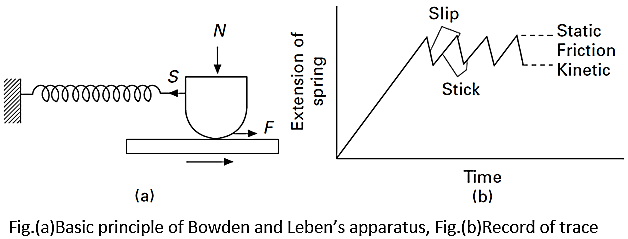Effect of friction in different stages of textile processing

Fiber stage:

→ Friction helps in drawing and drafting.

→ Frictional force holds together the fiber into yarn.

→ Fiber friction can lead to damage of fiber surface.

Yarn stage:

→ Friction helps to make yarn by twisting during spinning.

→ Friction causes nep formation.

→ Friction makes more clean yarn.

→ Friction increases yarn hairiness.

→ Uniform tension can be maintained during winding & warping  because of friction.

Fabric stage:

→ Friction increases lusture and smoothness of the yarn and the fabric.

→ Fabric feeling depends on different types of friction.

→ Low friction provides good fabric drape.

→ High friction causes low handle properties of fabric.

Factors affecting frictional force/intensity:

⦿  Number of contact point: Number of contact points­ Friction­

⦿  State of the surface: For harshness –  Friction­

For lubricated – Friction

Cleanliness  – Friction­

⦿  Cross sectional shape: For hexagonal fiber  –  Friction­

For round fiber   Friction

⦿  Temperature: Temperature­  Friction

⦿  Effect of water: Moisture regain­  Friction­

⦿   Fiber weight: Fiber wt.­   Friction­

⦿   Speed of sliding: Speed of sliding­    Friction­

⦿  Oil contact and viscosity: The frictional force increases both as the oil content is increased and as the viscosity of the oil increases.

LUBRICATION:

Polymers or fibers are lubricated with any suitable lubricant to prevent or minimize the effect of friction. Lubricants also prevents the static charges on the fibers, that affects the electrical circuits of the machine.

Effect of lubricant on friction

⦿  Lubricants are broadly used in the yarn and fiber production stages to reduce fiber-to-fiber and fiber-to-metal friction. A raw cotton on steel gave μ=0.25, whereas scoured cotton on steel gave μ = 0.7, and lubricated scoured cotton on steel gave values of μ ranging from 0.14 to 0.35.

⦿  Lubricants are applied to wool for a number of reasons associated with the friction characteristics of the scoured wool, but principally to minimize fiber breakage and static charge resulting from frictional contract with machine components.

⦿  Frictional force is increased when an excess amount of lubricant is used on the yarn surface. When excess oil contracted acetate yarn passes over a guide the frictional force is increased.

(2138)#### Md Sohanur Rahman Sobuj

Co-founder , Admin & Author at Textile Study Center
Student of Bangladesh University of Textiles (BUTEX) Department: Apparel Engineering#### Latest posts by Md Sohanur Rahman Sobuj (see all)Close
Share via